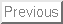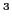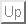# Continuity equation and local gauge invariance for the NLO nuclear Energy Density Functionals

F. Raimondi, B. G. Carlsson, J. Dobaczewski, J. Toivanen

November 11, 2011

### Abstract:

Background:
The next-to-next-to-next-to-leading order (NLO) nuclear energy density functional extends the standard Skyrme functional with new terms depending on higher-order derivatives of densities, introduced to gain better precision in the nuclear many-body calculations. A thorough study of the transformation properties of the functional with respect to different symmetries is required, as a step preliminary to the adjustment of the coupling constants.

Purpose:
Determine to which extent the presence of higher-order derivatives in the functional can be compatible with the continuity equation. In particular, to study the relations between the validity of the continuity equation and invariance of the functional under gauge transformations.

Methods:
Derive conditions for the validity of the continuity equation in the framework of time-dependent density functional theory. The conditions apply separately to the four spin-isospin channels of the one-body density matrix.

Results:
We obtained four sets of constraints on the coupling constants of the NLO energy density functional that guarantee the validity of the continuity equation in all spin-isospin channels. In particular, for the scalar-isoscalar channel, the constraints are the same as those resulting from imposing the standard U(1) local-gauge-invariance conditions.

Conclusions:
Validity of the continuity equation in the four spin-isospin channels is equivalent to the local-gauge invariance of the energy density functional. For vector and isovector channels, such validity requires the invariance of the functional under local rotations in the spin and isospin spaces.Next: introduction
Jacek Dobaczewski 2011-11-11